Smartick is an advanced online program that teaches kids math and coding in only 15 min. a day
Try it for free!

Feb13

# Multiplication with Decimals and Some Examples

Have you learned how to multiply with decimals yet? Today we are going to review three different cases of multiplication with decimals.

### Multiplication with decimals and whole numbers

In this case, we are multiplying a decimal by another number without decimals, as in the example: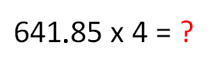• Step 1: We place both numbers so that the longer factor is on the top and the shorter factor is on the bottom.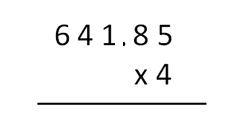• Step 2: We solve the multiplication problem as we normally would with whole numbers.  Afterward, we count the digits that come after the decimal point in the decimal and we place the decimal point in the answer so that it has the same number of decimal places after it as in the decimal in the factor position.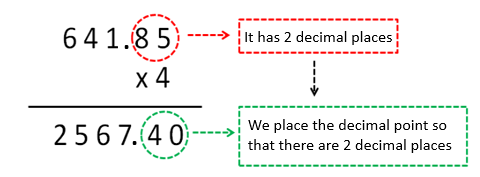### Multiplication when both factors are decimals

In this case, both factors are decimals: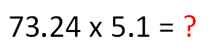• Step 1: As in the previous case, the first thing we have to do is to place the numbers so that the longer factor is on the top and the shorter factor is on the bottom.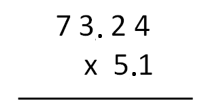• Step 2: We solve the multiplication problem as we normally would with whole numbers.  Afterward, we count the digits that come after the decimal points in both factors.  The answer should have as many decimal places as can be found in both factors combined.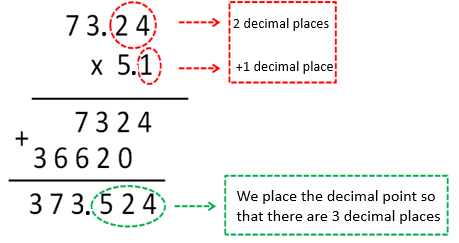### Multiplication with decimals and a whole number ending in zero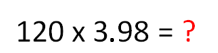In this case, the whole number factor ends in zero.

• Step 1: We break down the number into another number multiplied by 10: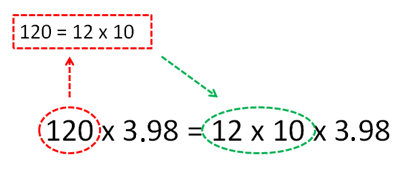• Step 2: We multiply the decimal by 10 (thereby getting rid of a decimal place).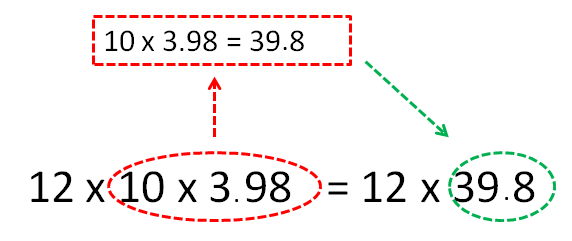• Step 3: We place the numbers and now we can multiply a decimal number by a whole number.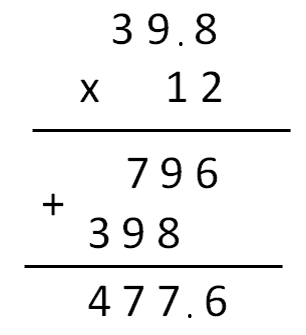Multiplication with decimals is easy, isn’t it?

If you want to continue learning math with Smartick while having fun, click on this link to sign up for a free trial.

Learn More:

Latest posts by Smartick (see all)

2 Comments

• Johnson HopeJul 06 2019, 4:49 PM

This was really helpful. Thanks a lot

Reply
• Nkosiey BJan 25 2019, 7:20 AM

this is great stuff. very helpful

Reply